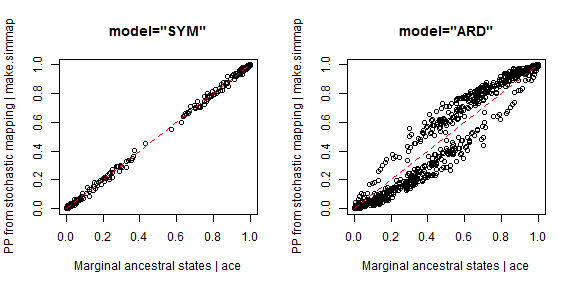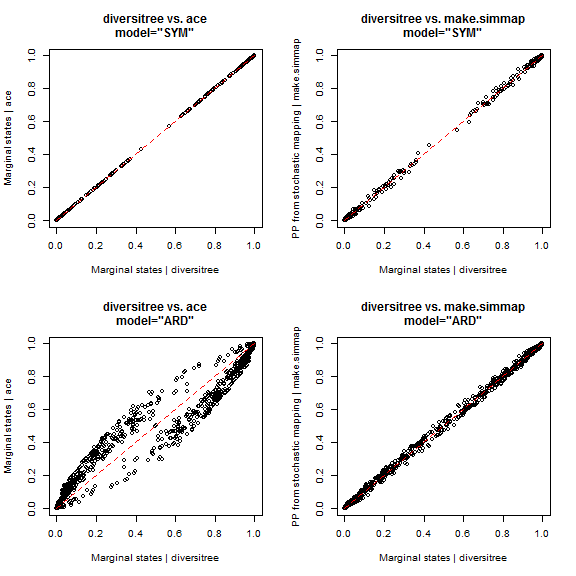## Wednesday, September 21, 2016

### Possible bug in `ace(...,model="ARD")` (and other non-symmetric models)

There appears to be a bug with `ace(...,model="ARD")`. Reversible models work fine with `ace`, ape's function for ancestral state estimation; however with asymmetric models - in which qi,j and qj,i are not necessarily equal for all i & j - something seems to be awry.

I will report this to Emmanuel, of course - but I thought that I would post the demo so that he (& others) can see what I mean.

I'm going to use an empirical dataset that was shared with me to help identify the problem; however I have removed all identifying information from the phylogeny & trait data. Note that the issue is not always nearly as readily identifiable as it is in this case. Note also that it does not have to do with model fitting, as the same non-reversible model is fit (more or less - to a reasonable standard of numerical precision) by `ace`, `make.simmap`, and diversitree. Rather the issue seems to be in the calculation of the marginal ancestral states given a particular fitted model.

``````library(phytools)
library(diversitree)

## SYM model
trees.SYM<-make.simmap(phy,x,model="SYM",nsim=500) ## make simmap
``````
``````## make.simmap is sampling character histories conditioned on the transition matrix
##
## Q =
##              0            1
## 0 -0.005818548  0.005818548
## 1  0.005818548 -0.005818548
## (estimated using likelihood);
## and (mean) root node prior probabilities
## pi =
##   0   1
## 0.5 0.5
``````
``````## Done.
``````
``````smp.SYM<-summary(trees.SYM) ## PP from stochastic mapping
fit.SYM<-ace(x,phy,type="discrete",model="SYM") ## marginal ASR

## ARD model
trees.ARD<-make.simmap(phy,x,model="ARD",nsim=500) ## make.simmap
``````
``````## make.simmap is sampling character histories conditioned on the transition matrix
##
## Q =
##              0            1
## 0 -0.026275656  0.026275656
## 1  0.008346216 -0.008346216
## (estimated using likelihood);
## and (mean) root node prior probabilities
## pi =
##   0   1
## 0.5 0.5
``````
``````## Done.
``````
``````smp.ARD<-summary(trees.ARD) ## PP from stochastic mapping
fit.ARD<-ace(x,phy,type="discrete",model="ARD") ## marginal ASR

## compare each one
par(mfrow=c(1,2))
plot(fit.SYM\$lik.anc,smp.SYM\$ace,main="model=\"SYM\"",
xlab="Marginal ancestral states | ace",
ylab="PP from stochastic mapping | make.simmap")
lines(c(0,1),c(0,1),lty="dashed",col="red")
plot(fit.ARD\$lik.anc,smp.ARD\$ace,main="model=\"ARD\"",
xlab="Marginal ancestral states | ace",
ylab="PP from stochastic mapping | make.simmap")
lines(c(0,1),c(0,1),lty="dashed",col="red")
``````These should both be the same - on average, that is. Stochastic mapping is, as the name implies, a stochastic procedure, so we might expect small differences. On average, and over a large number of replicates (as in this case; however) they should match.

It's hard to say what's wrong, here, so I thought I'd compare to diversitree which can also fit the Mk model & compute ancestral states:

``````## now compare both to diversitree
lik<-make.mk2(phy,x) ## make likelihood function
## first "SYM"
lik.sym<-constrain(lik,q01~q10)
fitted.sym<-find.mle(lik.sym,x.init=0.1)
asr.sym<-asr.marginal(lik.sym,pars=fitted.sym\$par)
## next "ARD"
fitted.ard<-find.mle(lik,x.init=c(0.1,0.1)) ## also tried root=ROOT.FLAT
asr.ard<-asr.marginal(lik,pars=fitted.ard\$par)

## compare back to ace & make.simmap
par(mfrow=c(2,2))
plot(t(asr.sym),fit.SYM\$lik.anc,
main="diversitree vs. ace\nmodel=\"SYM\"",
xlab="Marginal states | diversitree",
ylab="Marginal states | ace")
lines(c(0,1),c(0,1),lty="dashed",col="red")
plot(t(asr.sym),smp.SYM\$ace,
main="diversitree vs. make.simmap\nmodel=\"SYM\"",
xlab="Marginal states | diversitree",
ylab="PP from stochastic mapping | make.simmap")
lines(c(0,1),c(0,1),lty="dashed",col="red")
plot(t(asr.ard),fit.ARD\$lik.anc,
main="diversitree vs. ace\nmodel=\"ARD\"",
xlab="Marginal states | diversitree",
ylab="Marginal states | ace")
lines(c(0,1),c(0,1),lty="dashed",col="red")
plot(t(asr.ard),smp.ARD\$ace,
main="diversitree vs. make.simmap\nmodel=\"ARD\"",
xlab="Marginal states | diversitree",
ylab="PP from stochastic mapping | make.simmap")
lines(c(0,1),c(0,1),lty="dashed",col="red")
``````So it seems that `make.simmap` and diversitree agree for `model="ARD"` (remember, `make.simmap` is a stochastic method, so we don't expect them to match exactly, except as our number of simulations going to infinity), but that `ace` does not. That's all I can say at the moment about this, but it is probably worth looking into!

For full disclosure, this issue (identified as a possible error with `make.simmap`) was discovered by someone else & pointed out to me. (Also, initially I didn't believe it!)

1.Hi Liam. My question probably relates to the bug mentioned here.I am trying to run fitARD and its working fine for traits with less than four states but when I am doing it for those with 4 or more states, its giving me the following warning. I am using version 0.7.70 of Phytools. Is there any solution for this? Thanks!

Warning messages:
1: In nlminb(rep(ip, length.out = np), function(p) dev(p), ... :
2.